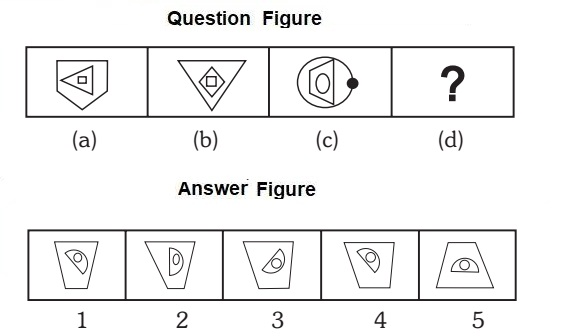Home » Non Verbal Reasoning » Analogy » Question

#### Analogy

Direction: Each of the following questions consists of two sets of figures. Figures (a), (b), (c) and (d) constitute the Question Set while figures (1), (2), (3), (4) and (5) constitute the Answer Set. There is a definite relationship between figures (a) and (b). Figure (a) and (b) are related in a particular way or manner. Establish the same relationship between (c) and (d).

1. Select a suitable figure from the answer figures that would replace the question mark (?) from question figure.1. 1
2. 2
3. 3
4. 4
5. 5
##### Correct Option: A

The outer most element reduces its side and inverted. The middle element moves 90 degree anti – clock wise and moves to outer side and the inner one moves to middle position at 45 degree anti clock wise.